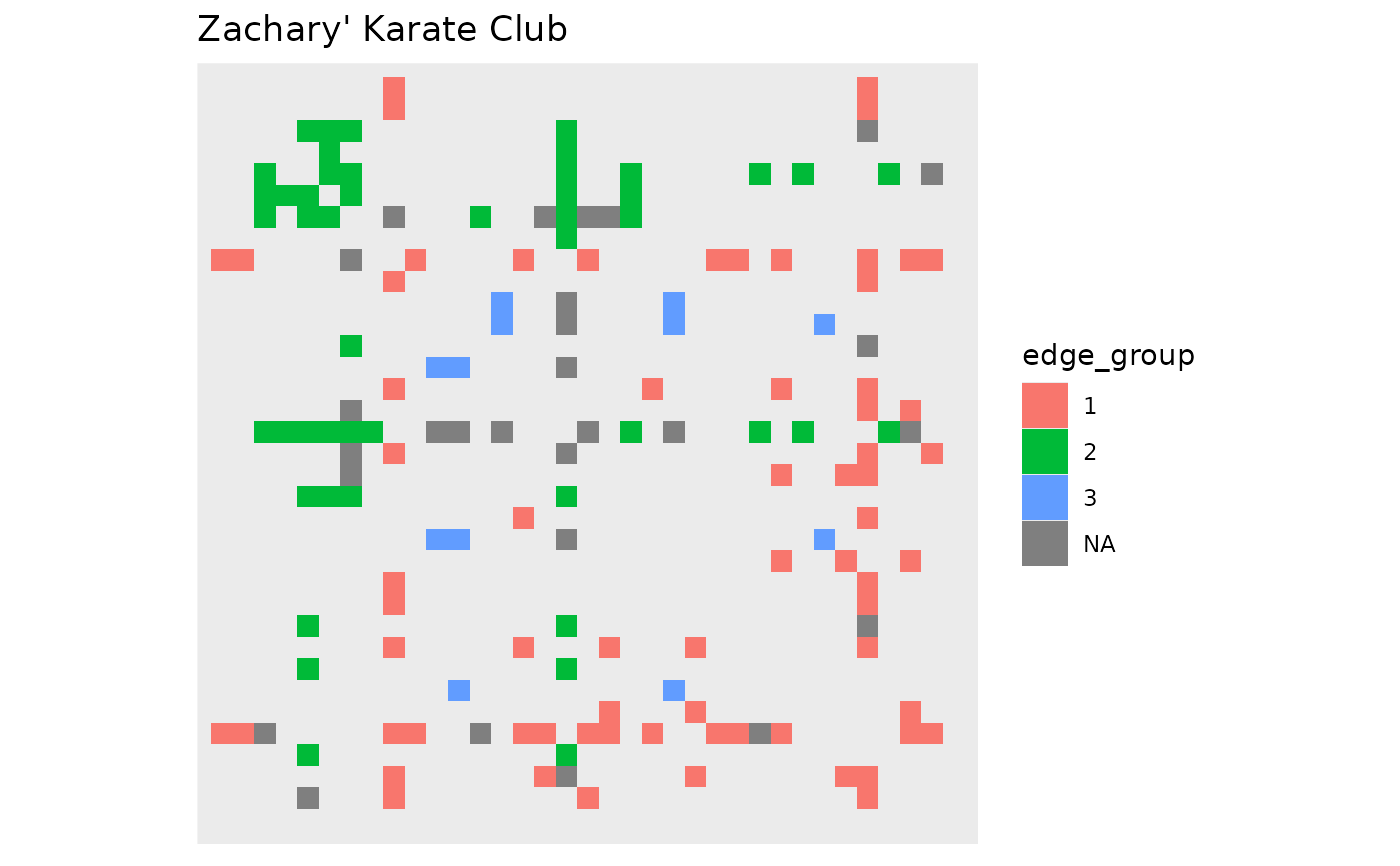This geom draws edges as tiles with their x-position defined by the x-position of the start node, and the y-position defined by the y-position of the end node. As such it will result in a matrix layout when used in conjunction with layout_tbl_graph_matrix()

geom_edge_tile(
mapping = NULL,
data = get_edges(),
position = "identity",
mirror = FALSE,
show.legend = NA,
...
)

## Arguments

mapping Set of aesthetic mappings created by ggplot2::aes() or ggplot2::aes_(). By default x, y, xend, yend, group and circular are mapped to x, y, xend, yend, edge.id and circular in the edge data. The return of a call to get_edges() or a data.frame giving edges in correct format (see details for for guidance on the format). See get_edges() for more details on edge extraction. Position adjustment, either as a string, or the result of a call to a position adjustment function. Logical. Should edge points be duplicated on both sides of the diagonal. Intended for undirected graphs. Default to FALSE logical. Should this layer be included in the legends? NA, the default, includes if any aesthetics are mapped. FALSE never includes, and TRUE always includes. It can also be a named logical vector to finely select the aesthetics to display. Other arguments passed on to layer(). These are often aesthetics, used to set an aesthetic to a fixed value, like colour = "red" or size = 3. They may also be parameters to the paired geom/stat.

## Aesthetics

geom_edge_tile understands the following aesthetics. Bold aesthetics are automatically set, but can be overridden.

• x

• y

• edge_fill

• edge_colour

• edge_size

• edge_alpha

• filter

## Edge aesthetic name expansion

In order to avoid excessive typing edge aesthetic names are automatically expanded. Because of this it is not necessary to write edge_colour within the aes() call as colour will automatically be renamed appropriately.

Other geom_edge_*: geom_edge_arc(), geom_edge_bend(), geom_edge_density(), geom_edge_diagonal(), geom_edge_elbow(), geom_edge_fan(), geom_edge_hive(), geom_edge_link(), geom_edge_loop(), geom_edge_parallel(), geom_edge_point(), geom_edge_span()

## Examples

require(tidygraph)
gr <- create_notable('zachary') %>%
mutate(group = group_infomap()) %>%
morph(to_split, group) %>%
activate(edges) %>%
mutate(edge_group = as.character(.N()\$group)) %>%
unmorph()#> Subsetting by nodes
ggraph(gr, 'matrix', sort.by = node_rank_hclust()) +
geom_edge_tile(aes(fill = edge_group), mirror = TRUE) +
scale_y_reverse() +
coord_fixed() +
labs(edge_colour = 'Infomap Cluster') +
ggtitle("Zachary' Karate Club")